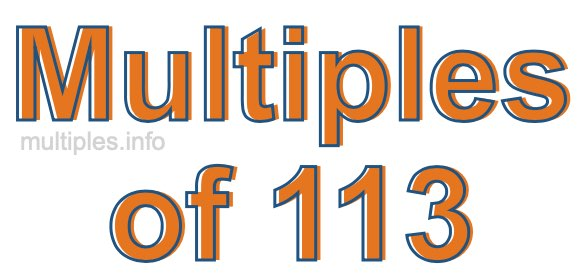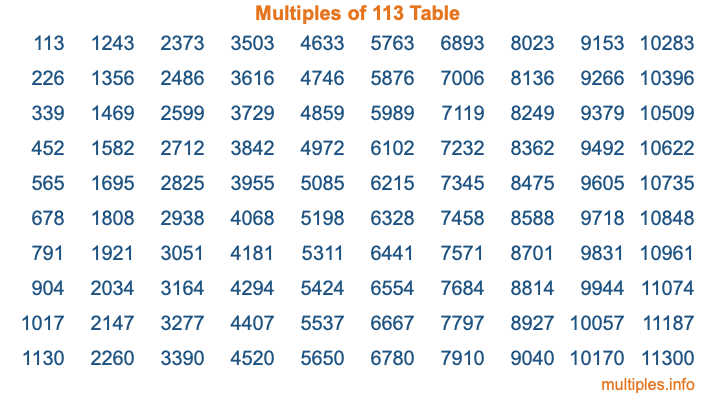Multiples of 113Welcome to the Multiples of 113 page. Here we will first teach you everything you will ever need to know about the multiples of 113, and then give you a study guide summary of everything we taught you to make sure you remember it all. Use this page to look up facts and learn information about the multiples of 113. This page will make you a multiples of one hundred thirteen expert!

Definition of Multiples of 113
Multiples of 113 are all the numbers that when divided by 113 equal an integer. Each of the multiples of 113 are called a multiple. A multiple of 113 is created by multiplying 113 by an integer.

Therefore, to create a list of multiples of 113, you start with 1 multiplied by 113, then 2 multiplied by 113, then 3 multiplied by 113, and so on for as long as you want. Thus, the list of the first five multiples of 113 is 113, 226, 339, 452, and 565. To see a larger list of multiples of 113, see the printable image of Multiples of 113 further down on this page. We also have a category where you can choose any nth multiple of 113.

Multiples of 113 Checker
The Multiples of 113 Checker below checks to see if any number of your choice is a multiple of 113. In other words, it checks to see if there is any number (integer) that when multiplied by 113 will equal your number. To do that, we divide your number by 113. If the the quotient is an integer, then your number is a multiple of 113.

Is  a multiple of 113?

Least Common Multiple of 113 and ...
A Least Common Multiple (LCM) is the lowest multiple that two or more numbers have in common. This is also called the smallest common multiple or lowest common multiple and is useful to know when you are adding our subtracting fractions. Enter one or more numbers below (113 is already entered) to find the LCM.

Check out our LCM Calculator if you need more details about the Least Common Multiple or if you need the LCM for different numbers for adding and subtraction fractions.

nth Multiple of 113
As we stated above, 113 is the first multiple of 113, 226 is the second multiple of 113, 339 is the third multiple of 113, and so on. Enter a number below to find the nth multiple of 113.

th multiple of 113

Multiples of 113 vs Factors of 113
113 is a multiple of 113 and a factor of 113, but that is where the similarities end. All postive multiples of 113 are 113 or greater than 113. All positive factors of 113 are 113 or less than 113.

Below is the beginning list of multiples of 113 and the factors of 113 so you can compare:

Multiples of 113: 113, 226, 339, 452, 565, etc.

Factors of 113: 1, 113

As you can see, the multiples of 113 are all the numbers that you can divide by 113 to get a whole number. The factors of 113, on the other hand, are all the whole numbers that you can multiply by another whole number to get 113.

It's also interesting to note that if a number (x) is a factor of 113, then 113 will also be a multiple of that number (x).

Multiples of 113 vs Divisors of 113
The divisors of 113 are all the integers that 113 can be divided by evenly. Below is a list of the divisors of 113.

Divisors of 113: 1, 113

The interesting thing to note here is that if you take any multiple of 113 and divide it by a divisor of 113, you will see that the quotient is an integer.

Multiples of 113 Table
Below is an image of the first 100 multiples of 113 in a table. The table is in chronological order, column by column. The first column has the first ten multiples of 113, the second column has the next ten multiples of 113, and so on.The Multiples of 113 Table is also referred to as the 113 Times Table or Times Table of 113. You are welcome to print out our table for your studies.

Negative Multiples of 113
Although not often discussed or needed in math, it is worth mentioning that you can make a list of negative multiples of 113 by multiplying 113 by -1, then by -2, then by -3, and so on, to get the following list of negative multiples of 113:

-113, -226, -339, -452, -565, etc.

Multiples of 113 Summary
Below is a summary of important Multiples of 113 facts that we have discussed on this page. To retain the knowledge on this page, we recommend that you read through the summary and explain to yourself or a study partner why they hold true.

There are an infinite number of multiples of 113.

A multiple of 113 divided by 113 will equal a whole number.

113 divided by a factor of 113 equals a divisor of 113.

The nth multiple of 113 is n times 113.

The largest factor of 113 is equal to the first positive multiple of 113.

113 is a multiple of every factor of 113.

113 is a multiple of 113.

A multiple of 113 divided by a divisor of 113 equals an integer.

113 divided by a divisor of 113 equals a factor of 113.

Any integer times 113 will equal a multiple of 113.

Multiples of a Number
Here you can get the multiples of another number, all with the same attention to detail as we did for multiples of 113 on this page.

Multiples of
Multiples of 114
Did you find our page about multiples of one hundred thirteen educational? Do you want more knowledge? Check out the multiples of the next number on our list!